# MCQs on Power Systems

##### Page 18 of 67. Go to page 1 2 3 4 5 6 7 8 9 10 11 12 13 14 15 16 17 18 19 20 21 22 23 24 25 26 27 28 29 30 31 32 33 34 35 36 37 38 39 40 41 42 43 44 45 46 47 48 49 50 51 52 53 54 55 56 57 58 59 60 61 62 63 64 65 66 67
01․ Bundled conductors are used for EHV transmission lines primarily for reducing the
corona loss
copper loss
voltage drop across the line
surge impedance of the line

Corona loss ∝ (Vph - Vc)² Where, Vph = Operating voltage of the transmission line Vc = Critical disruptive voltage Therefore, if Vc is more, corona loss will be less. Vc ∝ r Where, r = radius of the conductor Bundled conductors increases effective radius of the conductor and due to this, critical disruptive voltage will increase. Therefore, corona loss decreases by using of bundled conductors.

02․ Which of the following statements is true? Corona loss increases with
decrease in conductor size and decrease in supply frequency
increase in conductor size and decrease in supply frequency
decrease in conductor size and increase in supply frequency
increase in conductor size and increase in supply frequency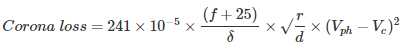Where, f = Supply frequency δ = Air density factor r = Radius of the conductor d = Distance between the conductors Vph = Operating voltage of the transmission line Vc = Critical disruptive voltage Therefore, Corona loss ∝ supply frequency and Corona loss ∝ (Vph - Vc)² Vc ∝ r Therefore, corona loss increases with the decrease in conductor size and increase in supply frequency.

03․ The corona loss on a particular system at 50 Hz is 1 kW/km per phase. What is the corona loss at 60 Hz in kW/km per phase?
1
1.25
0.89
1.13Where, f = Supply frequency δ = Air density factor r = Radius of the conductor d = Distance between the conductors Vph = Operating voltage of the transmission line Vc = Critical disruptive voltage Therefore,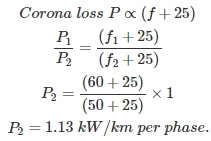04․ A 1- phase transmission line operating at 30 kV has radius of 1 cm and distance between conductors is 0.5 m. Assume m = δ =1, g = 21 kV/cm and frequency f = 50 Hz. Find the critical disruptive voltage?
17.32 kV/phase
34.64 kV/phase
82.15 kV/phase
41.075 kV/phase

Where, m = Surface factor δ = Air density factor r = Radius of the conductor d = Distance between the conductors g = Dielectric strength of air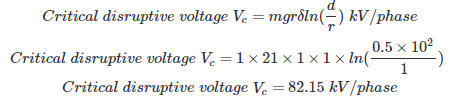05․ Which of the following statements is/are true? Due to corona,
interference increases
power loss increases
charging current increases
all of the above

Corona is the electrical discharge of atmospheric air. If the operating voltage is more than dielectric strength of atmospheric air, air molecules are ionized and start accumulating on the conductor surfaces producing corona. The effects of corona are 1. Interference increases 2. Power loss increases 3. Charging current increases

06․ Critical disruptive voltage is a voltage at which
corona is just initiated
corona is visible with blue or violet colour
both 1 and 2
nether 1 nor 2

The voltage at which corona is just initiated is called critical disruptive voltage(Vc). At this critical disruptive voltage, corona is not visible but noise can be heard.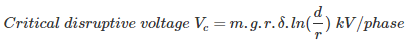Where, m = Surface factor δ = Air density factor r = Radius of the conductor d = Distance between the conductors g = Dielectric strength of air

07․ Which of the following statements is/are true? Corona is reduced by
increasing the radius of the conductor
increasing the distance between the conductor
decreasing the supply frequency
all of the aboveWhere, f = Supply frequency δ = Air density factor r = Radius of the conductor d = Distance between the conductors Vph = Operating voltage of the transmission line Vc = Critical disruptive voltage Therefore, Corona loss ∝ supply frequency and Corona loss ∝ (Vph - Vc)² Vc ∝ r Vc ∝ ln(d) Therefore, corona loss will decrease by 1. Increasing the radius of the conductor 2. Increasing the distance between the conductor 3. Decreasing the supply frequency 4. Increasing the height of the tower 5. Using transposition

08․ Critical visual voltage is the voltage at which
corona is just initiated
corona is visible with blue or violet colour
both 1 and 2
none of the above

The voltage at which corona is visible with blue or violet colour is called critical visual voltage(Vv). Critical visual voltage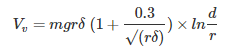Where, m = Surface factor δ = Air density factor r = Radius of the conductor d = Distance between the conductors g = Dielectric strength of air

09․ Which of the following statements is/are true?
Corona loss is more in HVAC system
Corona loss is more in HVDC system
same in both cases
none of the aboveWhere, f = Supply frequency δ = Air density factor r = Radius of the conductor d = Distance between the conductors Vph = Operating voltage of the transmission line Vc = Critical disruptive voltage Corona loss ∝ (f + 25) Therefore, corona loss is more in HVAC system compared to HVDC system.

10․ If the conductor diameter decreases, inductance of the line,
increases
decreases
remains unaffected
none of the above

The inductance of single phase two wire line is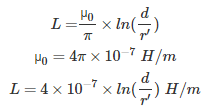Where, d = distance between the conductors r' = 0.7788 r r = radius of the conductor Therefore, If the conductor diameter decreases, inductance of the line increases.

<<<1617181920>>>